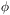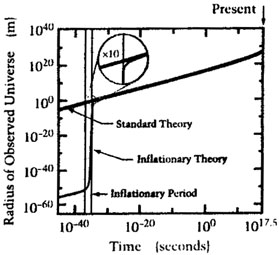### 3. THE INFLATIONARY UNIVERSE

Although the properties of the Big Bang are very special, we now know that the laws of physics provide a mechanism that produces exactly this sort of a bang. The mechanism is known as cosmic inflation.

The crucial property of physical law that makes inflation possible is the existence of states of matter that have a high energy density that cannot be rapidly lowered. Such a state is called a "false vacuum," where the word "vacuum" indicates a state of lowest possible energy density, and the word "false" is used to mean temporary. For a period that can be long by the standards of the early Universe, the false vacuum acts as if the energy density cannot be lowered, since the lowering of the energy is a slow process. The underlying physics of the false vacuum state is described in the box on the left.

Critical Mass Density and Flatness

THE CRITICAL MASS densityc related to the Hubble constant H byc = 3H2 / 8G

where G is Newton's gravitational constant). The quantityis defined by=/c, whereis the actual mass density. It is often assumed that the cosmological constantintroduced by Einstein is zero, in which case the Universe will recollapse if and only if> 1. Ifis non-zero, the condition for recollapse is more complicated, but the equation above is still taken as the definition ofc.

The spatial geometry of the Universe is determined by the quantity+/ 3H2

If this quantity exceeds one, the Universe curves back on itself to form a closed space of finite volume, but without boundary In such a space the sum of the angles in a triangle would exceed 180 degrees, and a starship traveling on a straight line would eventually return to its point of origin. If the quantity above is less than one, the Universe is an open space in which triangles contain less than 180 degrees. If the quantity is exactly one, the space is Euclidean, which is also called flat.

Physics of the False Vacuum

THE FALSE VACUUM arises naturally in any theory that contains scalar fields, that is, fields that resemble electric or magnetic fields except that they have no direction. The Higgs fields of the Standard Model of particle physics or the more speculative grand unified theories are examples of scalar fields. It is typical of Higgs fields that the energy density is minimal not when the field vanishes, but instead at some nonzero value of the field. For example, the energy density diagram might look like

The energy density is zero if=t, so this condition corresponds to the ordinary vacuum of empty space. In this context it is usually called the "true" vacuum. The state in which the scalar field is near= 0, at the top of the plateau, is called the "false" vacuum. If the plateau of the energy density diagram is flat enough, it can take a very long time, by early Universe standards, for the scalar field to "roll" down the hill of the energy density so that the energy can be lowered. For short times the false vacuum acts like a vacuum in the sense that the energy density cannot be lowered.

The peculiar properties of the false vacuum stem from its pressure, which is large and negative (see box on the right). Mechanically such a negative pressure corresponds to a suction, which does not sound like something that would drive the Universe into a period of rapid expansion. The mechanical effects of pressure, however, depend on pressure differences, so they are unimportant if the pressure is reasonably uniform. According to general relativity, however, there is a gravitational effect that is very important under these circumstances. Pressures, like energy densities, create gravitational fields, and in particular a positive pressure creates an attractive gravitational field. The negative pressure of the false vacuum, therefore, creates a repulsive gravitational field, which is the driving force behind inflation.

There are many versions of inflationary theories but generically they assume that some small patch of the early Universe somehow came to be in a false vacuum state Various possibilities have been discussed, including supercooling during a phase transition in the early Universe, or a purely random fluctuation of the fields. A chance fluctuation seems reasonable even if the probability is low, since the inflating region will enlarge by many orders of magnitude, while the non-inflating regions will remain microscopic. Inflation is a wildfire that will inevitably take over the forest, as long as there is some chance that it will start.

Once a patch of the early Universe is in the false vacuum state, the repulsive gravitational effect drives the patch into an inflationary period of exponential expansion. To produce a universe with the special features of the Big Bang discussed above, the expansion factor must be at least about 1025. There is no upper limit to the amount of expansion. Eventually the false vacuum decays, and the energy that had been locked in it is released. This energy produces a hot, uniform, soup of particles, which is exactly the assumed starting point of the traditional Big Bang theory. At this point the inflationary theory joins onto the older theory, maintaining all the successes for which the Big Bang theory is believed.

In the inflationary theory the Universe begins incredibly small, perhaps as small as 10-24 cm, a hundred billion times smaller than a proton. The expansion takes place while the false vacuum maintains a nearly constant energy density, which means that the total energy increases by the cube of the linear expansion factor, or at least a factor of 1075. Although this sounds like a blatant violation of energy conservation, it is in fact consistent with physics as we know it.

The resolution to the energy paradox lies in the subtle behavior of gravity. Although it has not been widely appreciated, Newtonian physics unambiguously implies that the energy of a gravitational field is always negative a fact which holds also in general relativity. The Newtonian argument closely parallels the derivation of the energy density of an electrostatic field, except that the answer has the opposite sign because the force law has the opposite sign: two positive masses attract, while two positive charges repel. The possibility that the negative energy of gravity could balance the positive energy for the matter of the Universe was suggested as early as 1932 by Richard Tolman, although a viable mechanism for the energy transfer was not known.

During inflation, while the energy of matter increases by a factor of 1075 or more, the energy of the gravitational field becomes more and more negative to compensate. The total energy - matter plus gravitational - remains constant and very small, and could even be exactly zero. Conservation of energy places no limit on how much the Universe can inflate, as there is no limit to the amount of negative energy that can be stored in the gravitational field.

This borrowing of energy from the gravitational field gives the inflationary paradigm an entirely different perspective from the classical Big Bang theory, in which all the particles in the Universe (or at least their precursors) were assumed to be in place from the start. Inflation provides a mechanism by which the entire Universe can develop from just a few ounces of primordial matter. Inflation is radically at odds with the old dictum of Democritus and Lucretius, "Nothing can be created from nothing" If inflation is right, everything can be created from nothing, or at least from very little. If inflation is right, the Universe can properly be called the ultimate free lunch.

Pressure of the False Vacuum

THE PRESSURE OF THE FALSE VACUUM can be determined by a simple energy-conservation argument. Imagine a chamber filled with false vacuum, as shown in the diagram below.

For simplicity, assume that the chamber is small enough so that gravitational effects can be ignored. Since the energy density of the false vacuum is fixed at some value uf, the energy inside the chamber is U=ufV, where V is the volume. Now suppose the piston is quickly pulled outward, increasing the volume by dV. If any familiar substance were inside the chamber, the energy density would decrease. The false vacuum, however, cannot rapidly lower its energy density, so the energy density remains constant and the total energy increases. Since energy is conserved, the extra energy must be supplied by the agent that pulled on the piston. A force is required, therefore, to pull the piston outward, implying that the false vacuum creates a suction, or negative pressure p. Since the change in energy is dU = ufdV, which must equal the work done, dW = -pdV, the pressure of the false vacuum is given by

p = -uf.

The pressure is negative, and extremely large. General relativity predicts that the gravitational field which slows the expansion of the universe is proportional to uf + 3p, so the negative pressure of the false vacuum overcomes the positive energy density to produce a net repulsive gravitational field.The solution to the horizon problem. The green line shows the radius of the region that evolves to become the presently observable Universe, as described by the traditional Big Bang theory. The black line shows the corresponding curve for the inflationary theory. Due to the spectacular growth spurt during inflation, the inflationary curve shows a much smaller Universe than in the standard theory for the period before inflation. The uniformity is established at this early time, and the region is then stretched by inflation to become large enough to encompass the observed Universe. Note that the numbers describing inflation are illustrative, as the range of possibilities is very large.